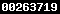# Error Propagation Calculator

This tool allows to determine the uncertainty (or error) of any mathematical expression that contains physical quantities with uncertainties. It follows the rules of the Gaussian error propagation: If f is a function of the independent variables X and Y, written as f(X,Y), then the uncertainty in f is obtained by taking the partial derivatives of f with respect to each variable, multiplication with the uncertainty in that variable, and addition of these individual terms in quadrature.

Use "." as decimal mark: 1.234, not 1,234.

Numerical stability is maintained for input values ranging from 1e-5 to 1e5 (see section "Notes" below).

## Instructions

1. Enter a valid formula using the functions listed at the bottom of this page.
2. In the "quantities with errors" section define all variables which appear in the formula. Use "." as decimal mark, not ",".
3. Click on "Evaluate" to obtain the result along with its absolute and relative uncertainty.

## ExampleFormula: log(a)+(b*pow(c,2))*sin(c) with variables a, b, c Exact error (calculated analytically): 56.88139881918965 Error calculated by this tool (numerically): 56.882447776373766 Deviation: 0.00104896 (≅ 0.02 ‰)

Check with Mathematica

## Notes

This tool is based on numerical methods. However, even for complicated formulas the deviation between numerical and analytical results is usually negligibly small. Please note that input values whose absolute is smaller than 1e-5 or larger than 1e5 in combination with can cause numerical instabilities. To manually adapt the step size used for the calculation of partial derivatives, overwrite the internal variable "hstep" by adding it to the "Quantities with errors" section. The standard value for hstep is 1e-7.

Write "pow(x,y)" instead of "^" or "**" for "raised to the power of". The following math methods are available and can be used in the formula field:

Method Description
acos(x) Returns the arccosine of x, in radians
asin(x) Returns the arcsine of x, in radians
atan(x) Returns the arctangent of x as a numeric value between -PI/2 and PI/2 radians
cos(x) Returns the cosine of x (x is in radians)
exp(x) Returns the value of Ex
log(x) Returns the natural logarithm (base E) of x
pow(x,y) Returns the value of x to the power of y
sin(x) Returns the sine of x (x is in radians)
sqrt(x) Returns the square root of x
tan(x) Returns the tangent of an angle

Euler's constant and Pi are represented by "E" and PI" respectively.Visitors: